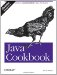# Recipe 5.16 Taking Logarithms

#### Problem

You need to take the logarithm of a number.

#### Solution

For logarithms to base e, use java.lang.Math 's log( ) function:

`// Logarithm.java  double someValue;  // compute someValue...  double log_e = Math.log(someValue);`

For logarithms to other bases, use the identity that:where x is the number whose logarithm you want, n is any desired base, and e is the natural logarithm base. I have a simple LogBase class containing code that implements this functionality:

`// LogBase.java  public static double log_base(double base, double value) {      return Math.log(value) / Math.log(base);  }`

#### Discussion

My log_base function allows you to compute logs to any positive base. If you have to perform a lot of logs to the same base, it is more efficient to rewrite the code to cache the log(base) once. Here is an example of using log_base:

`// LogBaseUse.java  public static void main(String argv[]) {      double d = LogBase.log_base(10, 10000);      System.out.println("log10(10000) = " + d);  }  C:> java LogBaseUse log10(10000) = 4.0`Java Cookbook, Second Edition
ISBN: 0596007019
EAN: 2147483647
Year: 2003
Pages: 409
Authors: Ian F Darwin

Similar book on Amazon